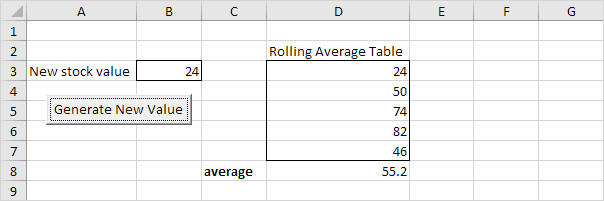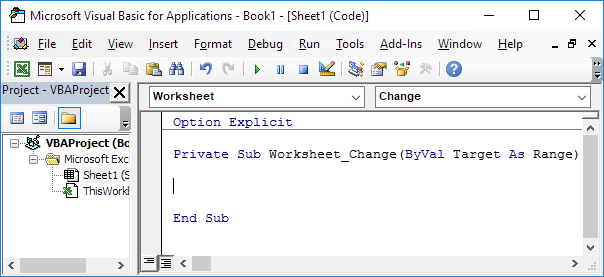# Rolling Average Table

Below we will look at a program in Excel VBA that creates a rolling average table. Place a command button on your worksheet and add the the following code line:

Range("B3").Value = WorksheetFunction.RandBetween(0, 100)

This code line enters a random number between 0 and 100 into cell B3. We want Excel VBA to take the new stock value and place it at the first position of the rolling average table. All other values should move down one place and the last value should be deleted.Create a Worksheet Change Event. Code added to the Worksheet Change Event will be executed by Excel VBA when you change a cell on a worksheet.

1. Open the Visual Basic Editor.

2. Double click on Sheet1 (Sheet1) in the Project Explorer.

3. Choose Worksheet from the left drop-down list. Choose Change from the right drop-down list.Add the following code lines to the Worksheet Change Event:

4. Declare a variable called newvalue of type Integer and two ranges (firstfourvalues and lastfourvalues).

Dim newvalue As Integer, firstfourvalues As Range, lastfourvalues As Range

5. The Worksheet Change Event listens to all changes on Sheet1. We only want Excel VBA to do something if something changes in cell B3. To achieve this, add the following code line:

6. We initialize newvalue with the value of cell B3, firstfourvalues with Range("D3:D6") and lastfourvalues with Range("D4:D7").

newvalue = Range("B3").Value
Set firstfourvalues = Range("D3:D6")
Set lastfourvalues = Range("D4:D7")

7. Now comes the simple trick. We want to update the rolling average table. You can achieve this by replacing the last four values with the first four values of the table and placing the new stock value at the first position.

lastfourvalues.Value = firstfourvalues.Value
Range("D3").Value = newvalue

8. Don't forget to close the if statement.

End if

9. Finally, enter the formula =AVERAGE(D3:D7) into cell D8.

10. Test the program by clicking on the command button.

Go to Next Chapter: Array